Precalculus : Determine if a Relation is a Function

Example Questions

Example Question #1 : Determine If A Relation Is A Function

Which of the following expressions is not a function?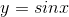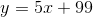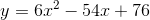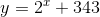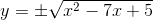Explanation:

Recall that an expression is only a function if it passes the vertical line test. Test this by graphing each function and looking for one which fails the vertical line test. (The vertical line test consists of drawing a vertical line through the graph of an expression. If the vertical line crosses the graph of the expression more than once, the expression is not a function.)

Functions can only have one y value for every x value. The only choice that reflects this is:Example Question #2 : Determine If A Relation Is A Function

Suppose we have the relation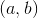on the set of real numbers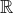whenever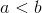. Which of the following is true.

The relation is a function because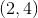holds and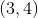also holds.

The relation is not a function because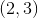andboth hold.

The relation is a function because every relation is a function, since that's how relations are defined.

The relation is not a function because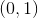holds but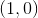does not.

The relation is a function because for every, there is only onesuch thatholds.

The relation is not a function becauseandboth hold.

Explanation:

The relation is not a function becauseandhold. If it were a function,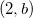would hold only for one. But we know it holds for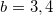because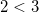and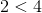. Thus, the relationon the set of real numbersis not a function.

Example Question #3 : Determine If A Relation Is A Function

Consider a family consisting of a two parents, Juan and Oksana, and their daughters Adriana and Laksmi. A relationis true wheneveris the child of. Which of the following is not true?

If the two parents had only one daughter, the relation would be a function.

(Laksmi,Adriana) does not hold because Adriana is not Laksmi's child.

Even if the two parents had only one daughter, the relation would not be a function.

The relation is not a function because (Laksmi,Juan) and (Laksmi,Oksana) both hold.

(Adriana,Laksmi) does not hold because Laksmi is not Adriana's child and is a boy.

Even if the two parents had only one daughter, the relation would not be a function.

Explanation:

The statement

"Even if the two parents had only one daughter, the relation would not be a function."

is not true because if they had only one daughter, say Adriana, then the only relations that would exist would be (Juan, Adriana) and (Oksana,Adriana), which defines a function.

Example Question #4 : Determine If A Relation Is A Function

Which of the following relations is not a function?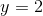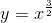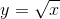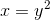Explanation:

The definition of a function requires that for each input (i.e. each value of), there is only one output (i.e. one value of). For, each value ofcorresponds to two values of(for example, when, bothand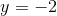are correct solutions). Therefore, this relation cannot be a function.

Example Question #5 : Determine If A Relation Is A Function

Given the set of ordered pairs, determine if the relation is a function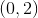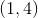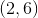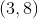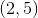Cannot be determined

No

Yes

No

Explanation:

A relation is a function if no single x-value corresponds to more than one y-value.

Because the mapping fromgoes toandthe relation is NOT a function.

Example Question #6 : Determine If A Relation Is A Function

What equation is perpendicular to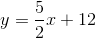and passes throgh?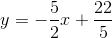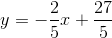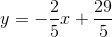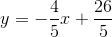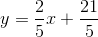Explanation:

First find the reciprocal of the slope of the given function.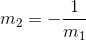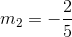The perpendicular function is: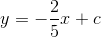Now we must find the constant,, by using the given point that the perpendicular crosses.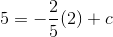solve for: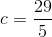Example Question #1 : Determine If A Relation Is A Function

Is the following relation of ordered pairs a function?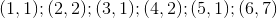Yes

Cannot be determined

No

Because there are no more than one correspondingvalue for any givenvalue, the relation of ordered pairs IS a function.